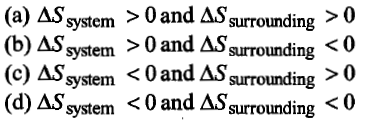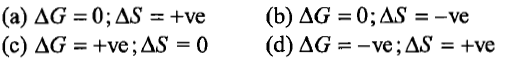The maximum work done in expanding 16 g oxygen at 300 K and occupying a volume of 5 dm3 isothermally until the volume becomes 25 dm3 is:

(a) 2.01 x 103 J                       (b) +2.81 x 103 J

(c) 2.01 x 10-3 J                      (d) +2.01 x 10-6 J

Concept Questions :-

Internal Energy and work done
High Yielding Test Series + Question Bank - NEET 2020

Difficulty Level:

1 mole of an ideal gas at 25$°\mathrm{C}$ is subjected to expand reversibly ten times of its initial volume. The change in entropy of expansion is:

(a) 19.15 JK-1mol-1                          (b) 16.15 JK-1mol-1

(c) 22.15 JK-1mol-1                          (d) none of these

High Yielding Test Series + Question Bank - NEET 2020

Difficulty Level:

For the process

H2O(l) $\to$H2O(g)

at T=100$°$C and 1 atmosphere pressure, the correct choice is:Concept Questions :-

Spontaneity and Entropy
High Yielding Test Series + Question Bank - NEET 2020

Difficulty Level:

(a) pressure is maintained constant

(b) gas is isothermally expanded

(c) there is perfect heat insulation

(d) the system changes heat with surroundings

Concept Questions :-

Internal Energy and work done
High Yielding Test Series + Question Bank - NEET 2020

Difficulty Level:

Heat of combustion $∆\mathrm{H}°$ for C(s), H2(g) and CH4(g) are -94, -68 and -213 kcal/mol. Then, $∆\mathrm{H}°$ for

C(s) + 2H2(g) $\to$CH4(g) is

(a) -17 kcal/mol                          (b) -111 kcal/mol

(c) -170 kcal/mol                        (d) -85 kcal/mol

Concept Questions :-

Thermochemistry
High Yielding Test Series + Question Bank - NEET 2020

Difficulty Level:

If 50 calorie are added to a system and system does work of 30 calorie on surroundings, the change in internal energy of system is:

(a) 20 cal                             (b) 50 cal

(c) 40 cal                             (d) 30 cal

Concept Questions :-

Enthalpy and It's Type
High Yielding Test Series + Question Bank - NEET 2020

Difficulty Level:

For the process:

H2O(l)[1 bar, 373 K] $\to$H2O(g)[1 bar, 373 K] the correct set of thermodynamic parameters are:Concept Questions :-

Spontaneity and Entropy
High Yielding Test Series + Question Bank - NEET 2020

Difficulty Level:

The internal energy change when a system goes from state A to B is 40 kJ/mol. If the system goes from A to B by a reversible path and returns to state A by an irreversible path. What would be the change in internal energy?

(a) 40 kJ                                    (b) >40 kJ

(c) <40 kJ                                  (d) Zero

Concept Questions :-

Internal Energy and work done
High Yielding Test Series + Question Bank - NEET 2020

Difficulty Level:

Change in entropy is negative for:

(a) Bromine (l)$\to$Bromine(g)

(b) C(s) + H2O(g) $\to$CO(g) + H2(g)

(c) N2(g,10 atm)$\to$N2(g,1 atm)

(d) Fe ( 1mol, 400 K) $\to$ Fe( 1mol, 300 K)

Concept Questions :-

Spontaneity and Entropy
High Yielding Test Series + Question Bank - NEET 2020

Difficulty Level:

The mathematical form of the first law of thermodynamics when heat (q) is supplied and W is work done by the system (+ve) is:

(a) $∆$U=q+W                        (b) $∆$U=q-W

(c) $∆$U=-q+W                       (d) $∆$U= -q-W

Concept Questions :-

Internal Energy and work done